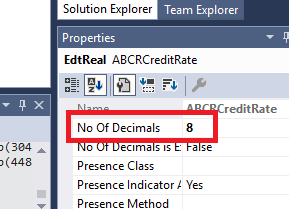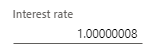## 20 Ağustos 2021 Cuma

### Dynamics 365 F&O - Extend decimal point beyond five

Dynamics 365 F&O supports six decimal points. So just extend decimal point field  of extended data type doesn't enough:We need to do a few tricks more:

In first we create a new field for save decimal point and be sure this method below to run after every write (We can call from update/insert methods).

public void updateInterestRateDecimals()
{
this.InterestRateDecimal = (this.InterestRate - real2int(this.InterestRate) ) *
100000000;
}

Than we modify postLoad like this:

{
super();
if (this.InterestRateDecimal != 0)
{
this.InterestRate = real2int(this.InterestRate) +
this.InterestRateDecimal / 100000000;
}
}

Result: